# Batch Normalization and ReLU for solving Vanishing Gradients

A logical and sequential roadmap to understanding the advanced concepts in training deep neural networks.

# Agenda

We will break our discussion into 4 logical parts that build upon each other. For the best reading experience, please go through them sequentially:

1. What is Vanishing Gradient? Why is it a problem? Why does it happen?
2. What is Batch Normalization? How does it help in Vanishing Gradient?
3. How does ReLU help in Vanishing Gradient?
4. Batch Normalization for Internal Covariate Shift

## 1.1 What is vanishing gradient?

First, let’s understand what vanishing means:

Vanishing means that it goes towards 0 but will never really be 0.

Vanishing gradient refers to the fact that in deep neural networks, the backpropagated error signal (gradient) typically decreases exponentially as a function of the distance from the last layer.

In other words, the useful gradient information from the end of the network fails to reach the beginning of the network.

## 1.2 Why is it a problem?

❓The crucial question at this stage is: why is it a problem if the initial starting layers of the network receive a very small gradient?

To understand this, recollect what is the role of a “gradient”? Well, a gradient is just the measure of how much the output variable changes for a small change in the input. And this gradient is then used to update/learn the model parameters — weights and biases. Below is the parameter updation rule typically followed:

Coming back to the issue at hand — what will happen if the derivative term in the above equation is too small, i.e- almost zero? We can see that a very small derivative would update or change the value of Wx only by a minuscule amount and hence the (new) Wx* would be almost equal to the (older) Wx. In other words, no change has been made to the model weights. And no change in the weights means no learning. The weights of the initial layers would continue to remain unchanged (or only change by a negligible amount), no matter how many epochs you run with the backpropagation algorithm. This is the problem of vanishing gradients!

Next, we move on to understand the mathematical reasoning of why vanishing gradients take place.

## 1.3 Why vanishing gradient happens?

❓The crucial question at this stage is: why do the initial starting layers of the network receive a very small gradient? Why do the gradient values diminish or vanish as we travel back into the neural network?

Vanishing gradients usually happen while using the Sigmoid or Tanh activation functions in the hidden layer units. Looking at the function plot below, we can see that when inputs become very small or very large, the sigmoid function saturates at 0 and 1 and the tanh function saturates at -1 and 1. In both these cases, their derivatives are extremely close to 0. Let’s call these ranges/regions of the function saturating regions or bad regions.

Thus, if your input lies in any of the saturating regions, then it has almost no gradient to propagate back through the network.

# 2. Batch Normalization

As the name suggests, batch normalization is some kind of a normalization technique that we are applying to the input (current) batch of data. Omitting the rigorous mathematical details, batch normalization can be simply visualized as an additional layer in the network that normalizes your data (using a mean and standard deviation) before feeding it into the hidden unit activation function.

But how does normalizing the inputs prevent vanishing gradients? It’s now time to connect the dots!

## 2.1 Batch Normalization for Vanishing Gradients

❓The crucial question at this stage is: How does normalizing the inputs ensure that the initial layers of the network do not receive a very small gradient?

Batch normalization normalizes the input and ensures that|x| lies within the “good range” (marked as the green region) and doesn’t reach the outer edges of the sigmoid function. If the input is in the good range, then the activation does not saturate, and thus the derivative also stays in the good range, i.e- the derivative value isn’t too small. Thus, batch normalization prevents the gradients from becoming too small and makes sure that the gradient signal is heard.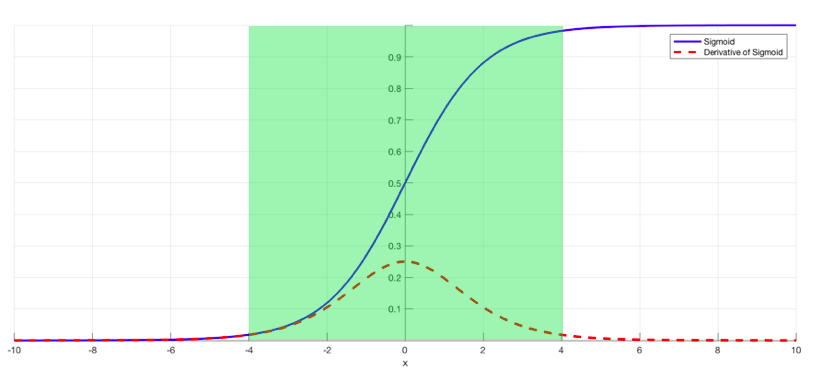Image 2: The “good range” of a sigmoid activation function (Source)

Now, although the gradients have been prevented from becoming too small, the gradients are still small because they always lie between [0,1]. Specifically, the derivate of sigmoid ranges only from [0, 0.25], and the derivative of tanh ranges only from [0, 1]. What could be an implication of this?

To get an answer, recollect the steps involved in training a deep neural network:

• Backpropagation finds the derivatives of the network by moving layer by layer from the final layer to the initial one.
• The gradient update of any layer using backpropagation consists of a number of multiplied gradients (due to the chain rule) accumulated over the layers from the end to the current layer.
• The further you get towards the start of the network, the more of these gradients are multiplied together to get the gradient update.
• The gradients values are typically in the range [0,1]. (As discussed above)
• Hence, if we multiply a bunch of terms that are less than 1, the more terms we have, the more the gradient value will tend towards zero.
• This issue is amplified and more serious for the initial layers of a neural network because a lot of these small gradients have been multiplied on the way (from end to start).

Thus, batch normalization alone cannot solve the problem of vanishing gradients when using with sigmoid and tanh.

# 3. ReLU for Vanishing Gradients

We saw in the previous section that batch normalization + sigmoid or tanh is not enough to solve the vanishing gradient problem. We need to use batch normalization with a better activation function — ReLU!

What makes ReLU better for solving vanishing gradients?

a) It does not saturate

b) It has constant and bigger gradients (as compared to sigmoid and tanh)

Below is a comparison of the gradients of sigmoid, tanh, and ReLU.

ReLU has gradient 1 when input > 0, and zero otherwise. Thus, multiplying a bunch of ReLU derivatives together in the backprop equations has the nice property of being either 1 or 0. There is no “vanishing” or “diminishing” of the gradient. The gradient travels to the bottom layers either as is or it becomes exactly 0 on the way.

# 4. Batch Normalization for Internal Covariate Shift

There is another reason why batch normalization works. The original batch normalization paper claimed that batch normalization was so effective in increasing the deep neural network performance because of a phenomenon called “Internal Covariate Shift”.

According to this theory, the distribution of the inputs to hidden layers in a deep neural network changes erratically as the parameters of the model are updated during backprop.

Since one layers’ outputs act as inputs for the next layer, and the weights are also being continuously updated for every layer through backprop — this means that the input data distribution of every layer is also constantly changing.
Using batch normalization, we limit the range of this changing input data distribution by fixing a mean and variance for every layer. In other words, the input to each layer is now not allowed to shift around much — constrained by a mean and variance. This weakens the coupling between the layers.

--

--

--

## More from Analytics Vidhya

Analytics Vidhya is a community of Analytics and Data Science professionals. We are building the next-gen data science ecosystem https://www.analyticsvidhya.com

## Solving Traveling Salesman Problem with SBM (Simulated Bifurcation Machine)## AISaturdayLagos: Basic Overview of CNN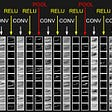## Cell instance segmentation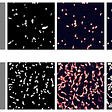## The Essential Guide to Building a Twitter Sentiment Analysis System## How to make a Pickle## Over-fit Model Vs Under-fit Model## Recognizing Handwritten Digits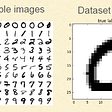## From Human Vision to Computer Vision — Convolutional Neural Network(Part3/4)## Lavanya Gupta

AWS ML Specialist | Instructor & Mentor for Data Science

## 5 questions to the core developer## Things to remember before using Machine Learning Algorithms## Neural Style Transfer Guide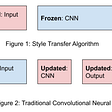## Actions over time speak louder than a transaction — Fraud detection using Machine Learning